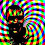## Sunday, February 8, 2009

### Unblurring Images

Tikhonov regularization is a pretty cool concept when applied to image processing. It lets you 'de-blur' an image, which is an ill-posed problem. The results won't be as spectacular as CSI, but still cool nonetheless.

### OriginalThe original image, CSI fans pretend it is a license plate or a criminal's face in a low quality surveillance video, was made by taking a screenshot of some words in a word processor. The image doesn't have to be character based, but characters make good practice problems.

### Blurring

We then blur the image with a Toeplitz matrix. In Octave that's pretty easy:

[m, n] = size(im);

sigma_true = 10;
p = exp(-(0:n-1).^2 / (2 * sigma_true^2))';
p = p + [0; p(n:-1:2)];
p = p / sum(p);

G_true = toeplitz(p);

im = G_true*double(im)*G_true';Generally after doing some floating point operations on an image you won't end up with something still scaled between 0 and 255, so the little function shown below rescales a vector's values to the proper range for a grayscale image.

function im = image_rescale(double_vector)
%% scale values linearly to lie between 0 and 255
m = max(double_vector);
n = min(double_vector);
scale = max(double_vector) - min(double_vector);
im = double_vector - (min(double_vector) + scale/2);
im = im ./ scale;
im = (255 .* im) + 127.5;
endfunction

### De-Blurring

This is where the regularization comes in. Since the problem is poorly conditioned we can't just recover the image by straightforward inversion. We'll use the singular value decomposition of our blurring matrix to create a regularized inverse, this is similar to the pseudoinverse. If the singular value decomposition of the blurring matrix isThen the regularized inverse isWhere the entries in the diagonal matrix are given bySo as alpha approaches zero we approach the un-regularized problem. Chapter 4 of Watkins has good coverage of doing SVDs in Matlab. The Octave to perform this process is shown below in the function tik_reg().

function im_tik = tik_reg(im,sigma,alpha)
[m,n] = size(im);
p = exp(-(0:n-1).^2 / (2 * sigma^2))';
p = p + [0; p(n:-1:2)];
p = p / sum(p);

G = toeplitz(p);

[U, S, V] = svd(G,0);

Sinv = S / (S^2 + alpha*eye(length(S),length(S))^2);

im_tik = V*Sinv*U'*double(im)*V*Sinv*U';
endfunction

This gives us two parameters (sigma and alpha) we have to guess in a real image processing problem (because we won't be the ones blurring the original). Another weakness of the method is that we assumed we knew the model that produced the blur, if the real physical process that caused the blur is not similar to Gaussian noise, then we won't be able to extract the original image.

Here's a couple for-loops in Octave that try several combinations of alpha and sigma.

sigma = [9,10,11];
alpha = [1e-6,1e-7,1e-8];

for j = 1:length(sigma)
for i = 1:length(alpha)
unblur = tik_reg(im, sigma(j), alpha(i));
unblur = reshape(image_rescale(reshape(unblur,m*n,1)),m,n);
fname = sprintf("unblur_%d%d.png",i,j);
imwrite(fname, uint8(unblur));
endfor
endforOptical character recognition probably wouldn't work on the output, but a person can probably decipher what the original image said.

#### 1 comment:

1.